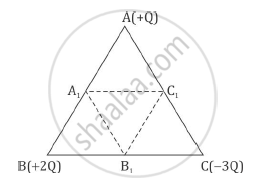# Three Point Charges, + Q + 2q and – 3q Are Placed at the Vertices of an Equilateral Triangle Abc of Side L. If These Charges Are Displaced to the Mid-point A1, B1 and C1, Respect - Physics

Sum

Three point charges, + Q + 2Q and – 3Q are placed at the vertices of an equilateral triangle ABC of side l. If these charges are displaced to the mid-point A1, B1 and C1, respectively, find the amount of the work done in shifting the charges to the new locations.#### Solution

q1= +Q

q2= +2Q

q3= -3Q

r = l (for each side)

Intial potential energy of system

U_1=1/(4piin_0l)[(q_1xxq_2)+(q_2xxq_3)+(q_3xxq_1)]

U_1=1/(4piin_0l)[(Qxx2Q)+(2Qxx(-3Q))+((-3Q) xxQ)]

U_1=(-7Q^2)/(4piin_0l

These charges displaced to mid points then final potential energy of system,

U_2=1/(4piin_0l/2)[(q_1xxq_2)+(q_2xxq_3)+(q_3xxq_1)]

U_2=2/(4piin_0l)[(Qxx2Q)+(2Qxx(-3Q))+((-3Q)xxQ)]

U_2=(-7Q^2)/(2piin_0l)

Work done, W = U2 - U1

W=(-7Q^2)/(2piin_0l)-(-7Q^2)/(4piin_0l)

W=(7Q^2)/(piin_0l)[(-1)/2-((-1)/4)]=(7Q^2)/(piin_0l)[(-1)/2+1/4]

W=(-7)/4(Q^2/(piin_0l))

Concept: Electrical Potential Energy of a System of Two Point Charges and of Electric Dipole in an Electrostatic Field
Is there an error in this question or solution?